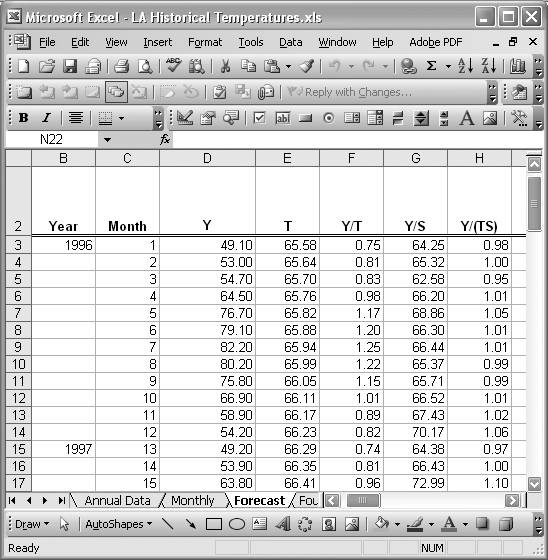# Deseasonalization of a Time Series

Problem

You're working with a time series with clear seasonal components, which you'd like to isolate and remove from the original data.

Solution

Discussion

In the previous recipe, I showed you how to compute seasonal indices for a time series using the average-percentage method. That recipe required nothing more than standard spreadsheet techniques to compute the indices. Further, the earlier Recipe 6.6 showed you how to use standard spreadsheet techniques to isolate and remove the long-term trend in a time series. The model used there was the multiplicative model in which the original time series, Y, is assumed to be composed of a long-term trend, seasonal variation, and irregular variation, which is represented in the formula Y = TSI. Deseasonalizing a time series amounts to estimating the S contribution and removing it by dividing Y by S (that is, by computing Y/S). You can extend these ideas further to isolate the irregular variation as well by computing Y/(TS) to yield I.

Let's reconsider the average monthly temperature series shown earlier in Figure 6-23. That data series has a slight upward trend and a very clear seasonal variation over each year. Using the seasonal indices computed in the previous recipe for this time series, we can easily decompose and deseasonalize the series. Figure 6-26 shows a spreadsheet I set up to decompose the time series.

Figure 6-26. Average monthly temperature decompositionColumn Y represents the original time series data. These are the average monthly temperatures plotted in Figure 6-23. This data series exhibits a slight upward trend, which can be modeled with the linear trendline equation T = 0.0592x + 65.521. Column T in Figure 6-26 computes this trend. Detrending this series is carried out as before (in Recipe 6.6), by dividing the original data Y by the trend T. Column Y/T contains the result.

Deseasonalization is carried out in a similar manner. However, here we divide the original series, Y, by the seasonal index for corresponding months. For example, the first cell in column Y/S, cell G3, contains the formula =D3/Jan. Jan is a name I defined for the January seasonal index computed in the previous recipe. Likewise, the formula in cell G4 for the month of February 1996 is =D4/Feb. I named all the seasonal indices in this manner and each monthly Y in each year is divided by the corresponding seasonal index. The results are contained in column Y/S and represent the deseasonalized series. Figure 6-27 shows the resulting deseasonalized temperature series.

Figure 6-27. Deseasonalized temperature seriesYou can now detrend the deseasonalized series by dividing every value in the Y/S column by the trend value in the T column. These calculations are carried out in the Y/(TS) column in Figure 6-26, where the cell formulas are of the form =G3/E3.

In some cases (e.g., if your data exhibits a significant long-term trend), you might prefer to detrend the data series first and then compute seasonal indices on the detrended series using the same techniques discussed in the previous recipe. Then seasonal components can be removed from the detrended series using the techniques discussed here. In either case, you've effectively isolated the irregular variation component, I, of the original series.

In Recipe 6.9, I'll show you how to extend the material presented in this and earlier recipes to predict the average monthly temperatures for the year 2000 based on the 1996 through 1999 historical data shown in Figure 6-23.Excel Scientific and Engineering Cookbook (Cookbooks (OReilly))
ISBN: 0596008791
EAN: 2147483647
Year: N/A
Pages: 206
Authors: David M Bourg

Similar book on Amazon